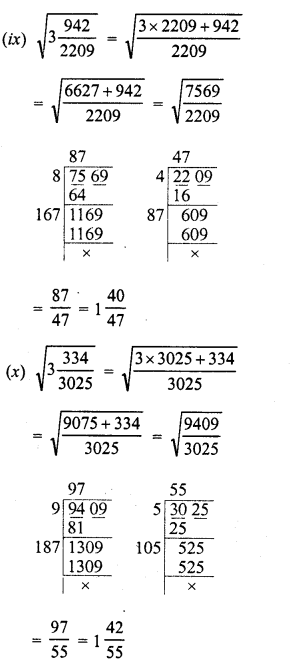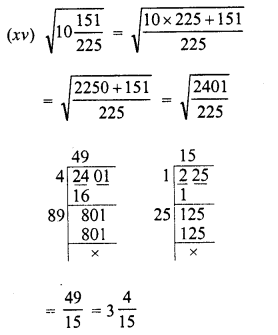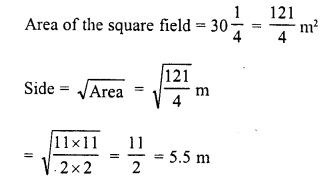# RD Sharma Class 8 Solutions Chapter 3 Squares and Square Roots Ex 3.6

In this chapter, we provide RD Sharma Class 8 Solutions Chapter 3 Squares and Square Roots Ex 3.6 for English medium students, Which will very helpful for every student in their exams. Students can download the latest RD Sharma Class 8 Solutions Chapter 3 Squares and Square Roots Ex 3.6 Maths pdf, free RD Sharma Class 8 Solutions Chapter 3 Squares and Square Roots Ex 3.6 Maths book pdf download. Now you will get step by step solution to each question.

# RD Sharma Class 8 Solutions Chapter 3 Squares and Square Roots Ex 3.6

Question 1.
Find the square root of:Solution:Question 2.
Find the value of :Solution:Question 3.
The area of a square field is 80 244729 square metres. Find the length of each side of the field.
Solution:Question 4.
The area of a square field is 30 14 m2. Calculate the length of the side of the squre.
Solution:Question 5.
Find the length of a side of a square playground whose area is equal to the area of a rectangular field of dimensions 72 m and 338 m.
Solution:
Length of rectangular field (l) = 338 m
and breadth (b) = 72 m
∴ Area = 1 x 6= 338 x 72 m2
∴ Area of square = 338 x 72 m2 = 24336 m2
and length of the side of the squareAll Chapter RD Sharma Solutions For Class 8 Maths

—————————————————————————–

All Subject NCERT Exemplar Problems Solutions For Class 8

All Subject NCERT Solutions For Class 8

*************************************************

I think you got complete solutions for this chapter. If You have any queries regarding this chapter, please comment on the below section our subject teacher will answer you. We tried our best to give complete solutions so you got good marks in your exam.

If these solutions have helped you, you can also share rdsharmasolutions.in to your friends.